Taking the ACT Math test can feel daunting: you have only 60 minutes to answer 60 questions, so it’s a good idea to be armed with some ACT Math strategies! If you’re wondering, “How can I improve my Math ACT score?” or “What math should I study for the ACT?” then this ACT Math study guide is for you. Our ACT Math tips are designed to give you the maximum strategic advantage as you take or retake this section of the ACT.

Looking for more general ACT study advice? You might like our articles on How to Improve Your ACT Score and ACT Test Strategies. Need winning strategies for the other ACT sections? Check out all of the articles in our series of ACT strategy guides:

## 1. Learn How the ACT Math Test is Structured

Our first ACT Math strategy is to make sure that you know exactly how the ACT math section is structured. Having a basic understanding of how this section is set up can help you better plan out how you want to spend your time as you take this section of the test.

• If you’re taking the ACT Math section as part of the full ACT, you will encounter it second, after the English section.
• The ACT Math section is arranged roughly by difficulty, with the easier questions at the beginning, and the most difficult questions occurring towards the end of the test.
• Questions 1-10 will generally be easier than questions 11-20 (and 11-20 easier than 21-30), but this does NOT mean that question 1 is easier than question 2.
• Despite the wide range of difficulty, each question is worth the same amount: 1 point.

Knowing this latter point is especially useful when it comes to budgeting your time. Spend the majority of your time on the earlier (easier) questions to ensure the most accuracy. Since harder questions are not worth more than easier ones, it makes sense to focus on earning as many points as possible from the questions that you know how to do.

If you’re taking the full ACT, you will get a short break after the Math section—knowing that you will soon have a chance to stretch, hydrate, use the restroom, and take a mental break should help you maintain your focus while taking the ACT Math test

Additionally, keep in mind that you are permitted to use a calculator on the ACT Math section. For specifics, you can read the ACT’s full calculator policy.

## 2. Familiarize Yourself with ACT Math Content

Next up on our list of ACT Math tips is getting yourself familiar with the types of ACT Math content you are going to encounter on this section of the ACT test. ACT Math questions fall into one of two large content areas: Integrating Essential Skills and Preparing for Higher Math.

We explain each content area briefly for the sake of keeping this strategy review succinct. If you feel you need more review of the ACT Math content, Piqosity’s 70+ ACT content lessons can help you master each type of ACT Math question, including information on all of the ACT Math formulas to know.

### Integrating Essential Skills

Integrating Essential Skills questions cover the math skills students should have learned before high school. Slightly less than half (40-43%) of the questions on the ACT Math section deal with mathematics concepts that most students should have learned before entering high school. These concepts include:

• Area, surface area, perimeter, and volume
• Proportions (including fractions and ratios)
• Rates and percentages
• Averages (mean, median, and mode)

Although the skills themselves are fairly straightforward, you may be asked to combine them or otherwise utilize them in increasingly complex ways.

### Preparing for Higher Math

Preparing for Higher Math questions cover subject matter that should have been learned in high school math and are split into five distinct subsections. A slight majority (57-60%) of the questions on the ACT Math section deal with “higher” mathematics concepts. Broadly speaking, these concepts deal with the math students learn once they have begun using algebra as a general way of expressing and solving equations.

These questions fall into five subcategories, listed in decreasing order of frequency in which they appear:

• Algebra: Students will be asked to solve a variety of expressions and equations, including those involving polynomials, exponents, radicals, and logarithms.
• Geometry: Students will be tested on their knowledge of shapes and solids. Questions will involve the concepts of congruence and similarity, as well as standard geometry rules involving angle relationships. Knowledge of trigonometric relationships and functions will also be applied in this section.
• Functions: These questions require the understanding and application of function notation, as well as knowledge of the graphs of linear, piecewise, radical, polynomial, and logarithmic functions.
• Statistics and Probability: Statistical concepts such as measures of central tendency, variance, and standard deviation will be tested. Students will also be asked to solve for probabilities and expected values.
• Number and Quantity: These questions require understanding of the various types of real and complex numbers. Materials that are tested under this category include exponents, vectors, and matrices.

## 3. Develop a Systematic Approach to Answering Questions

Even though every question on the ACT Math test is different (duh!), one of the most helpful ACT Math strategies is to develop a systematic approach that you can apply to every question. We recommend the following five-step method:

### 1. Ask: What concept is being tested?

First, determine what general concepts you will need to understand to correctly answer a question. Some questions will only involve one math concept, while others may require you to draw on a variety of mathematical knowledge.

For example, the following question (taken from the 2020 official ACT practice test) tests a single concept, ratios: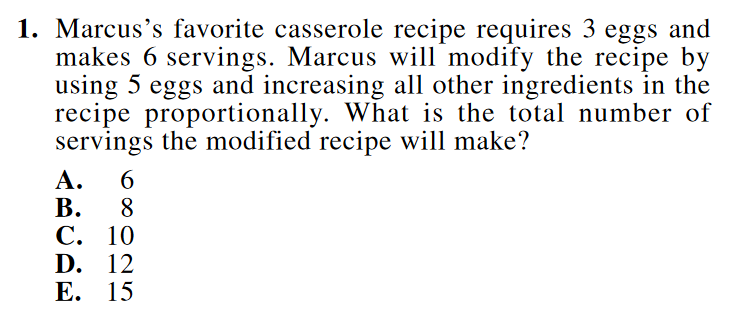To find the correct answer, we must find the relationship between the number of eggs and the number of servings, which is 3:6. Then we must find the answer which maintains an equivalent ratio when the number of eggs is increased to 5. The number of servings is double the number of eggs, so the correct answer is 10, choice C.

Sometimes, however, a question will test you on more than one concept. In the example below (taken from the same official practice test), the question requires you to understand both algebraic equations and fractions: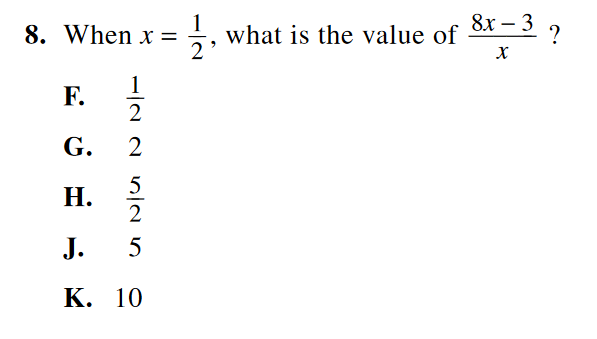First, you must use your knowledge of algebra and plug x=½ into the equation to get [8(½) – 3]/(½). Simplify to get [4-3]/(½), and again to get 1/(½). Now apply your knowledge of fractions:  division by a fraction is equivalent to multiplying the numerator by the reciprocal of the denominator. In this case, in order to divide 1 by ½, you must multiply 1 by the reciprocal of ½, which is 2. The correct answer is thus 2, choice G.

### 2. Use any related formulas.

After you’ve identified what concepts are required to answer a question, write down any formulas that are related to the tested concept. If you’re not sure exactly how to solve the problem, seeing the formulas in front of you may help you recognize where to plug in the given values to solve the problem.

If the tested concept does not involve any formulas, skip this step.

### 3. Review the given information.

Some of the ACT Math questions are presented as word problems; when you encounter one of these you will need to convert the words into an equivalent mathematical expression or equation.

Students often aren’t sure how to approach a word problem, so they tend to waste time by sitting there and staring at it. Unfortunately, the answer to a question doesn’t usually magically appear before your eyes. So one of our ACT math tips is to write down the information provided in the problem to help your brain see the problem in a new, often easier, way.

For example, the following problem (again from the same practice test) presents information about how two different book clubs charge their members. To solve this problem, the words will need to be converted into two algebraic equations, which you can then solve.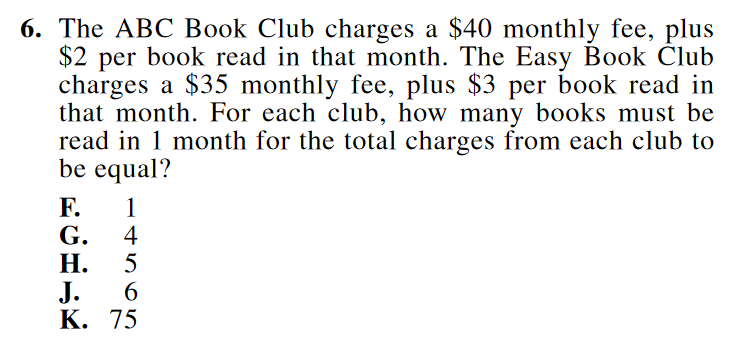The equation for the ABC Book Club might take the form y=2x+40, while the Easy Book Club equation might be written y=3x+35. Now, instead of a complicated word problem, you have a system of equations you can set equal to each other! (After doing this, you can determine that the answer is 5, choice H.)

### 4. Draw a picture.

In a similar vein, if the question describes a shape or image, but does not provide a visual representation, you should draw your own picture. Having a visual representation often makes it easier to understand what a question is asking you.

For example, the question below (from the ACT’s online practice problems) describes a situation but does not provide a picture of the situation.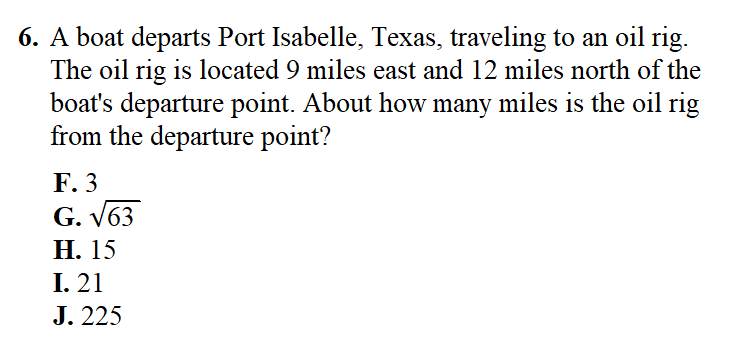Simply reading the question, you may incorrectly assume that you can determine the correct answer by adding the two distances together (9+12=21). However, after drawing a picture (like the one below), you can see that these lengths form a right triangle, and you will need to use the Pythagorean Theorem to solve the problem.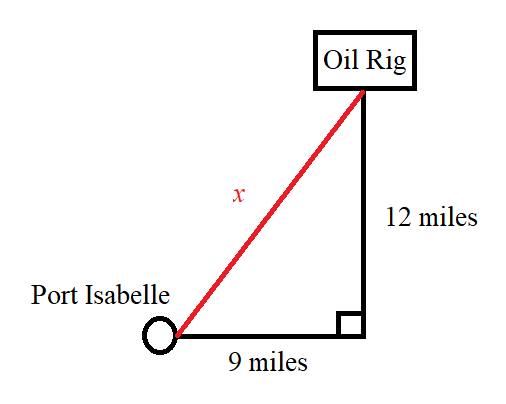Thus we can see that 92+122=x2. Solving this equation eventually leads us to x=15, answer choice H.

### 5. If all else fails, make an educated guess.

If you don’t understand the question after following these steps, or you feel that the question will take too long to answer, circle it and come back to it after you have completed all of the questions that you do know how to answer. If you have time left, you can try to work out the answer to the question, and if not, you should try to make an educated guess based on the information provided.

Let’s revisit the first example problem (about Marcus and his favorite casserole):Let’s say you don’t know how to answer this question, and you need to make an educated guess. You might notice that Marcus is almost (but not quite) doubling the recipe, which originally made 6 servings. As such, 12 servings would be too many (wrong answer), and 8 servings would be too little (wrong answer). The correct answer must be between these numbers: the only choice is 10!

## 4. Brush Up on Specific ACT Math Test Strategies

Once you’ve got a good grasp of the question types, it’s time to focus on a handful of ACT Math tips designed to help you with the actual process of taking the ACT Math section. Remember, taking a standardized test like the ACT is a skill, and as with all skills, practice makes perfect. These ACT Math strategies will help maximize your chances of success.

### Work backwards.

You already know that the correct answer is one of five choices. If you’re not sure how to solve the problem, see if you can “back solve” by plugging each answer choice into the original problem. (Note: Not every problem can be solved this way—this method works best if the answer choices are all integers.)

A related method, when dealing with questions that have a number of variables, is to substitute “easy” numbers for some or all of the variables, and work through the problem. “Easy” numbers are those which are straightforward to manipulate mathematically (like 1, 2, or 10).

### Use the figures.

When there is a graph, chart, or table involved in the problem, start there. If you can clearly understand what the figure is showing, you can use it to help solve any related questions. You can also sometimes use it to eliminate answers (for instance, if one answer choice is incompatible with a provided graph).

While all of the questions on the ACT Math Test can, in theory, be done without the use of a calculator, it can speed up or aid you in certain questions. But by the same token, using a calculator on a very easy question might actually be less efficient. If you can solve a problem easily on paper, don’t waste time plugging it into your calculator. Instead, save the calculator for harder questions like those testing geometry and trigonometry concepts, which usually appear in the last third of the ACT Math test.

## 5. Identify Your Specific ACT Math Test Weaknesses

Every student who takes the ACT Math test has their own set of test-taking strengths and weaknesses. As we’ll see momentarily in our section on strategic practice, in order to improve upon your particular weaknesses for the ACT Math section, you’ll need to be able to identify them first.

Common issues for students taking the ACT Math test include:

• Timing Issues: You scored very well on every question you answered, but didn’t have time to finish, you’ll know that what you need to work on most is increasing your test-taking speed.
• Application Issues: You are able to understand the content required to correctly answer each question, but struggle to identify what questions are asking. What you most need to work on is improving your understanding of how to apply all of your content knowledge.
• Content Issues: You have some underlying comprehension issues (for instance, maybe you don’t fully understand standard deviations) which make it difficult to answer certain questions. Your best tactic is to focus your study efforts on those content areas until you have a better understanding of them.

## 6. Practice Strategically

If you apply only one of our tips for how to improve your ACT Math score, this is the one you want to use. We truly can’t emphasize the importance of regular ACT practice enough. The more you practice, the more familiar you will be with the test, and the more likely you will get an outstanding score. But it’s not just a matter of rote repetition: if you’re thinking, “How do I study for the Math ACT?” the answer is—strategically!

We recommend the following 4-step plan to gain the maximum strategic advantage.

### 1. Take a full practice ACT Math test

After you’ve spent some significant time going over the content and studying ACT Math strategies, take a practice ACT Math section, like one of the two below:

We recommend trying to imitate real-life testing conditions. Clear your workspace of everything except your testing materials. Set a timer for 60 minutes, and work through as much of the test as you can; stop when the time’s up, even if you’re not done. As you’re taking the test, put a star next to any questions you guess on. When the timer goes off, stop and circle every question you didn’t have time to answer.

Mark each question “right” or “wrong.” For each question (even the ones you got right), read over the answer explanations. Go over each possible answer choice and learn why it is right or wrong. You will, of course, want to pay special attention to the questions you missed to better understand how you went wrong. Use the links below to access the answer explanations to previously published practice ACT Math tests:

### 3. Keep analyzing the data, finding your weak spots, and identifying solutions

As we covered above, recognizing your knowledge gaps and test taking weaknesses is a crucial part of figuring out how to improve your ACT Math score. As you continue to practice, you will want to continually assess whether your weaknesses are improving and whether you’ve identified new problem areas. Use your grading notes to draw conclusions about what you need to work on.

If your test taking weaknesses center around particular kinds of questions (as opposed to timing), Piqosity can help. Our ACT Answer Explanations categorize every question based on its type, helping you to get consistent and thorough practice. If your weakness is related to timing, our platform allows you to simulate a testing environment so that you can improve your performance in this area as well.

### 4. Target your weaknesses with practice

Once you know your weak spots, you’ll want to spend the majority of your study time focusing on those areas. This is where Piqosity’s 70+ content lessons and personalized practice options really come in handy—you can create practice tests which specifically target the areas where you most need improvement.

Of course, you want to make sure not to lose ground in your strongest subject areas. One helpful strategy is to divide your time more equally the closer you get to the test day. If, for instance, you had a month of prep time, you might spend the first week working exclusively on practice questions targeting your weakest content areas. In the second week you would continue working mostly in those areas, but by the end of the month you would spend equal time preparing for all of the content areas. (You might even take another full practice ACT Math test.)

Curious about the effectiveness of ACT prep? Read Piqosity’s deep-dive article: Does ACT Prep Work?

## Test Practice with Piqosity: The Ultimate in ACT Math Strategies

Now that you have these ACT math tips and strategies under your belt, it’s time to get studying! Whether you’re a student who wants to improve their ACT test taking skills, a teacher with multiple students wishing to give their scores a boost, or a tutoring company in need of a test prep LMS that really works, Piqosity has you covered with quality, affordable test prep materials.

Piqosity provides personalized practice test questions that help you address and improve upon your weaker test subject areas. Our software is engineered to help students study smarter, not harder. We offer a wide range of ACT prep materials, perfect for both tutored and self-guided ACT practice:

• 10 full-length ACT Practice tests
• Over 70 concept lessons, including tutorial videos
• Real-time Score prediction (both Composite and for each Subject)
• Interactive interface allowing teachers, tutors, or parents to assign and track student work
• …and much more!

The best part? Piqosity for the ACT is entirely free during the pandemic and is also typically available to try for free with our 7-day trial. Sign up today!

More Educational Resources by Piqosity: